# Rec. 2020 RGB to Spectrum Conversion for ReflectancesPublished October 1, 2018 by Scott Allen Burns, last updated May 25, 2020

Change Log
10/1/18 Initial publication.
10/2/18 Added section of object colors and XYZ color space.
11/3/19 Clarified some points and removed the XYZ space section.
1/7/20 Clarified that “real” colors are more commonly known as “object” colors.
1/8/20 Added section applying methods 2 and 3 and discussion of emissive sources.
1/17/20 Fixed a typo.
2/6/20 Fixed a typo. Clarified distinction between the triangular 2D chromaticity gamut and the 3D gamut (0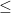rgb1).
5/25/20 Added link to Wiley Online Library in the January 7 update for the CR&A paper.

#### Introduction

In a previous presentation on generating reflectance curves from sRGB triplets, I presented several algorithms for creating a reasonably realistic spectral reflectance curve associated with a given sRGB color. This publication is a short note on applying the methods to a more recent RGB implementation called Recommendation ITU-R BT.2020-2 (10/2015), or Rec. 2020 for short.

#### Generating a New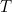Matrix

The colorimetric specification of this RGB system is:

The main task in applying the reflectance generating algorithms to Rec. 2020 is to create a newmatrix (called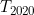here) that relates reflectance,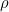, to linear Rec. 2020 rgb through the relationship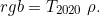Once this is done, just substituteforin any of the previous algorithms to make them specific to Rec. 2020. Recall thatis defined as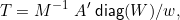where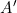is a 3×36 matrix of color matching functions (CMFs) that relate a 36×1 reflectance vector,, to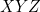tristimulus values.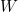is a 36×1 illuminant vector, which is Standard Illuminant D65 in this case. The expression diag() refers to a square 36×36 matrix withon the main diagonal and zeros elsewhere. The scalar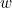is a normalizing factor that is calculated as the dot (scalar) product of the second row ofand. The matrix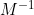here is the tricky part. It relatesto linear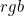and must be custom built for Rec. 2020.

Bruce Lindbloom has a handy page that describes the creation of M. The computation only requires the chromaticity coordinates of the RGB primaries and the tristimulus values of the reference white. For D65, these are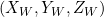= (0.95047, 1.00000, 1.08883).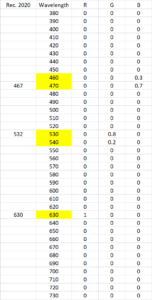I made one minor adjustment to the Rec. 2020 specs to compensate for the 10 nm intervals in our calculations. The R/G/B chromaticity coordinates shown in Table 3 above correspond to single wavelength primaries at 630 nm, 532 nm, and 467 nm, respectively. Since the last two wavelengths don’t fall on the 10 nm intervals, I modified them just slightly. The G primary is changed to be the combination of 80% 530 nm light and 20% 540 nm light. The B primary is changed to be 30% 460 nm light and 70% 470 nm light. This is shown in the table to the right (click to enlarge).

The chromaticity coordinates of the slightly modified primaries come out to be (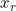,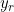) = (0.7079, 0.2920), (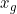,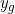) = (0.1718, 0.7941), and (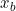,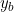) = (0.1312, 0.0478). This change greatly improves the stability of the optimization process (by avoiding slight constraint infeasibility very near the spectral envelope) and changes thevalues by only 0.00074 at most. Note that if a smaller wavelength interval is used to form thematrix, specifically 1 nm intervals, then this interpolating step is not necessary since the primaries at 467 nm, 532 nm, and 630 nm each align with a single wavelength band.

Plugging these values into the equations on Bruce Lindbloom’s page yields anmatrix of:We now have all the ingredients to build ourmatrix: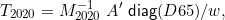which is available here.

Here are some results from applying a general-purpose optimization code (Matlab’s fmincon) to the nonlinear program (ILSS), usinginstead of the sRGB version of,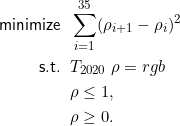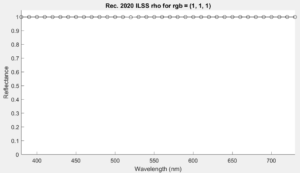As expected, a larger rgb of (1,1,1) produced a flat reflectance curve at 1.

I tried to use a highly saturated rgb, like (1,0,0), but the method failed (the optimizer reported it lost constraint feasibility). I used progressively smaller values for r until I found something that worked, rgb = (0.079, 0, 0). It ended up being a very dim, single wavelength spike at the Rec. 2020 r primary (630 nm).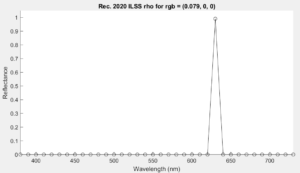The brightest purely saturated red I could find that worked in the optimizer.

Even though the optimizer was trying to find the reflectance curve with least slope squared, it had no recourse but to end up with a very non-smooth solution. This issue is addressed in the next section.

#### Rec. 2020 and “Object” Reflectances

UPDATE 1/21/20: The discussion above was working under the assumption that a reflectance curve in the range 0-1 was necessary. If we relax the upper bound, and only require that the reflectance is positive, then we have access to many more solutions. See the January 7, 2020 update below for further discussion on this matter. If the 0-1 bounds are desired, then continue reading this section.

When I originally developed the methods for converting sRGB values to reflectances, I was pleased to find that they worked for all values of sRGB. Furthermore, the two methods that guarantee reflectance curves in the range 0 to 1 produced a valid answer for all sRGB inputs. This will not be the case with Rec. 2020. Because the primaries are single wavelength lights, there are values of linear Rec. 2020 rgb triplets that have no corresponding reflectance curves in the range 0 to 1. Colors that can be represented by spectral reflectance distributions that vary between 0 and 1 are known as “object colors.”

Consider a reflectance curve with all zeros except at 630 nm, which is given a value of 1. This is the red primary in this color space. If we pre-multiply this reflectance by, we will get the Rec. 2020 rgb triplet corresponding to the reflectance. It comes out to be rgb = (0.0798, 0, 0). This reflectance represents a very dim, but extremely saturated red color. If we try to find a reflectance curve with a larger red rgb component, the only way that is possible is for the reflectance to exceed 1 in some places. This will manifest itself in the optimization methods as lost feasibility in the case of general-purpose optimization codes, or as bad conditioning (singular matrices) in the case of the tightly implemented algorithms like ILLSS, etc.

I was curious to find out just how much of the Rec. 2020 color space can be represented by reflectance curves with 0-1 range. I wrote a program that generated a large set of reflectance curves that contained only 1s and 0s, having one or two contiguous blocks of 1’s and 0s elsewhere. These are “optimal” colors that form the outer surface of the object color solid. All of these reflectance curves were then premultiplied byto get their corresponding rgb values, which were then plotted in Rec. 2020 color space. The Rec. 2020 rgb values that form the boundary of the object color solid are shown below in Rec. 2020 color space (roll mouse over to animate):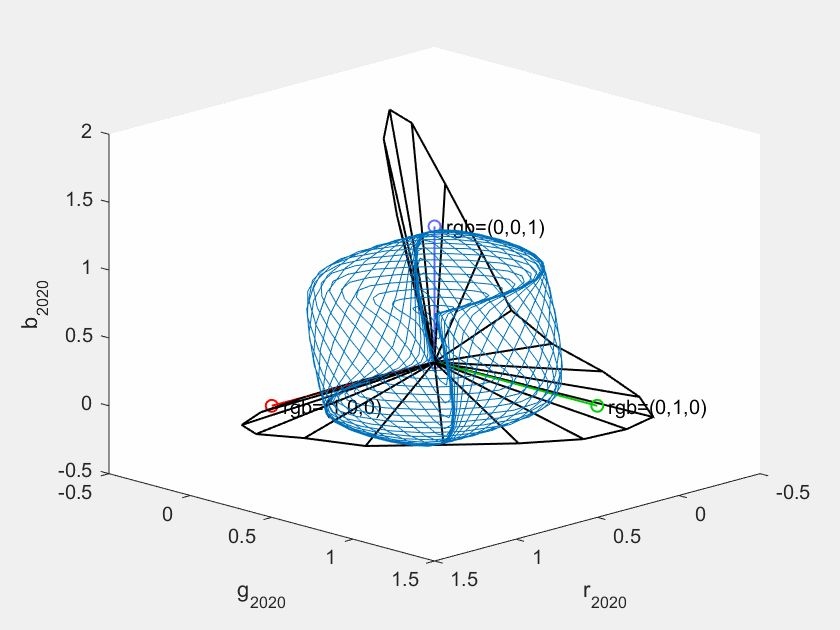Rec. 2020 color space, showing the spectral envelope (black) and the region of rgb values that can be represented by a reflectance curve with magnitudes between 0 and 1, i.e., object colors. The locations of rgb (1,0,0), (0,1,0), and (0,0,1) are also shown.

Here are some views from specific angles: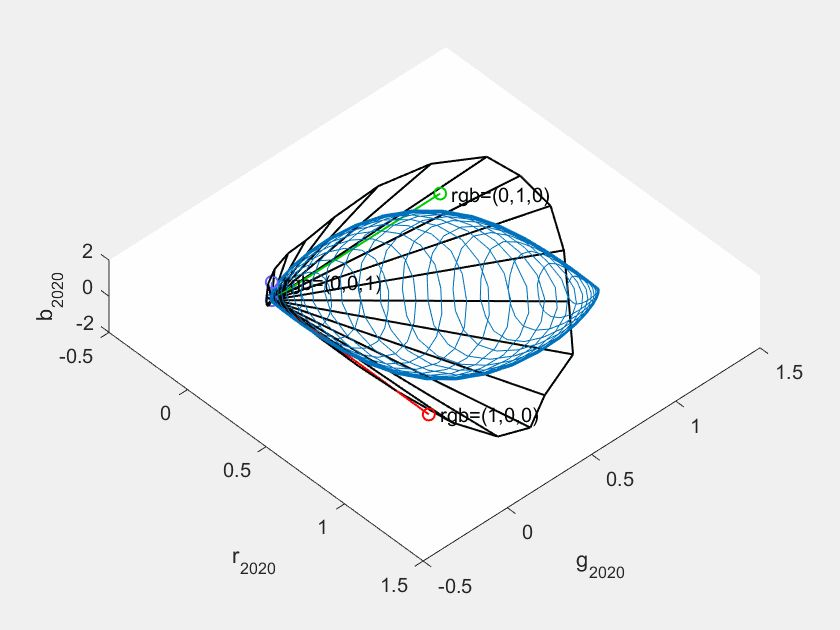Another angle of the rotation of axes gif (hover mouse over it to animate, click to enlarge).

The conclusion here is that if Rec. 2020 is intended to be used to generate reflectance reconstructions in the 0-1 range, an additional check should be performed to make sure the rgb values are within the object color boundary.

One final observation relates to the spiked reflectance curve for rgb = (0.079, 0, 0), above. If a tiny bit of another rgb component is added, i.e., rgb = (0.079, 0.079, 0), the nature of the curve changes dramatically: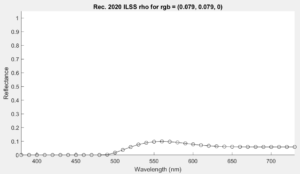Adding a tiny bit of another rgb component changes the spiked curve into a very smooth one.

Apparently, the additional green component pulls the color far enough away from the spectral envelope to allow the optimizer to find the broad, smooth spectrum reflectance curve it is seeking.

________________________________________

#### Update January 7, 2020

I’ve recently published a paper titled “Numerical Methods for Smoothest Reflectance Reconstruction,” (cited as “Burns SA. Numerical methods for smoothest reflectance reconstruction. Color Res Appl. 2020;45(1):8-21.”), which presents several of the methods found on the earlier web page in a more concise manner.

In a nutshell, the journal paper presents three of the methods for reconstructing reflectance curves from sRGB values, and recasts them in a form suitable for reconstructing reflectance curves from standard tristimulus values,. The three methods in the journal paper are called methods 1, 2, and 3. They are related to the webpage methods as:

Method 1: Applies the LSS (Least Slope Squared) method to generate reflectance curves from any XYZ values. It is very fast, but some curves may end up with reflectance values above or below the 0-1 range.

Method 2: Applies the LLSS (Least Log Slope Squared) method to generate a strictly positive reflectance curve from any XYZ values that fall within the spectral locus.

Method 3: Applies the LHTSS (Least Hyperbolic Tangent Slope Squared) method to generate a reflectance curve strictly between 0 and 1 for any XYZ values that fall within the object color solid.

It dawned on me that it would be very easy to apply methods 1-3 to the Rec. 2020 color space by simply substituting the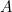matrix of color matching functions with thematrix developed above.

First let’s look at method 3 applied to Rec. 2020 space, which will give results very similar to what is presented above. Here is the MATLAB/Octave code for method 3, as it appears in the paper:


function [D,Aw]=method_3_prep(A,W)
% This function computes two arrays in preparation
% for method 3 (the object colors case).
% A is nx3 matrix of color matching functions.
% W is nx1 illuminant vector, scaled arbitrarily.
% D is nxn matrix of finite differencing constants.
% Aw is nx3 matrix of illuminant-W-referenced CMFs.
n=size(A,1);
D=full(gallery('tridiag',n,-2,4,-2));
D(1,1)=2; D(n,n)=2;
W_normalized=W/(A(:,2)'*W);
Aw=diag(W_normalized)*A;

 

and


function rho=method_3(D,Aw,XYZw)
% D is nxn matrix of finite differencing constants.
% Aw is nx3 matrix of illuminant-W-referenced CMFs.
% XYZw is a 3-element vector of illum-W-referenced
%      tristimulus values in the 0 <= Y <= 1 range.
% rho is nx1 reflectance vector (or zeros if failure).
n=size(Aw,1);
rho=zeros(n,1); z=zeros(n,1); lambda=zeros(3,1);
count=0; % iteration counter
maxit=20; % max number of iterations
ftol=1.0e-8; % convergence tolerance
while count <= maxit
r=(tanh(z)+1)/2;
d1=diag((sech(z).^2)/2);
d1a=d1*Aw;
d2=diag(sech(z).^2.*tanh(z));
F=[D*z+d1a*lambda; Aw'*r-XYZw(:)];
J=[D-diag(d2*Aw*lambda), d1a; d1a', zeros(3)];
delta=J\(-F); % solve J*delta = -F
z=z+delta(1:n);
lambda=lambda+delta(n+1:n+3);
if all(abs(F) < ftol) % convergence check
rho=(tanh(z)+1)/2; return
end
count=count+1;
end

 

Note that the code is broken up into two parts. The first part computes two arrays that depend only on the illuminant (D65 in this case). The second function constructs the reflectance curve from the illuminant-referenced tristimulus values,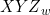. Normally, the first function would be called once, and then a series of reflectances would be computed by the second function. This is much more computationally efficient than recomputing the first two arrays over and over if we are considering only a single illuminant.

How do we apply this code to Rec. 2020 color space? Easy! Just usecomputed above in place of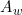, and use the Rec. 2020 rgb values in place of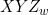. For example, the mauve color mentioned above (Rec. 2020 rgb=(0.7, 0.3, 0.5)) would be used to compute the reflectance reconstruction by the MATLAB commands:

>> D=full(gallery('tridiag',36,-2,4,-2));
>> D(1,1)=2; D(36,36)=2;
>> rho=method_3(D, T2020', [0.7,0.3,0.5]);

(Note that we don't need to computesince we already have. However, we do need to form the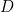matrix. Also note that the transpose ofis used since it is 3x36 andis 36x3.)

Method 3 has the same restrictions that were discussed above, that is, the rgb values must be within the object color solid in Rec. 2020 space. However, method 2 releases this restriction, as discussed below.

##### Generating reflectance curves for any Rec. 2020 rgb within its chromaticity gamut

Method 2 applies to anythat falls within the spectral locus. Consequently, since the primaries of Rec. 2020 space fall within the spectral locus, we should expect method 2 to work with any rgb triplet located within the triangular chromaticity gamut of the Rec. 2020 color space, including those with r>1, g>1, and/or b>1. Furthermore, method 2 will work with some colors outside the Rec. 2020 chromaticity gamut (i.e., having rgb values with some negative components) as long as the color is within the spectral locus.

Here is the code for method 2:


function rho=method_2(D,Aw,XYZw)
% D is nxn matrix of finite differencing constants.
% Aw is nx3 matrix of illuminant-W-referenced CMFs.
% XYZw is a 3-element vector of illum-W-referenced
%      tristimulus values in the 0 <= Y <= 1 range.
% rho is nx1 reflectance vector (or 0 if failure).
n=size(Aw,1);
rho=zeros(n,1); z=zeros(n,1); lambda=zeros(3,1);
count=0; % iteration counter
maxit=20; % max number of iterations
ftol=1.0e-8; % convergence tolerance
while count <= maxit
r=exp(z); dr=diag(r); dra=dr*Aw; v=dra*lambda;
F=[D*z+v; Aw'*r-XYZw(:)];
J=[D+diag(v), dra; dra', zeros(3)];
delta=J\(-F); % solve J*delta = -F
z=z+delta(1:n);
lambda=lambda+delta(n+1:n+3);
if all(abs(F) < ftol) % convergence check
rho=exp(z); return
end
count=count+1;
end


Here are some reconstructed reflectances from key points in the Rec. 2020 gamut, and the MATLAB code that generated the curves and their plots:

white, rgb=(1,1,1)
>> rho=method_2(D, T2020', [1,1,1]);
>> plot([380:10:730],rho); xlabel('Wavelength (nm)'); ylabel('Reflectance'); title('White'), ylim([0,1.1])red, rgb=(1,0,0)
>> rho=method_2(D, T2020', [1,EPS,EPS]);
>> plot([380:10:730],rho); xlabel('Wavelength (nm)'); ylabel('Reflectance'); title('Red')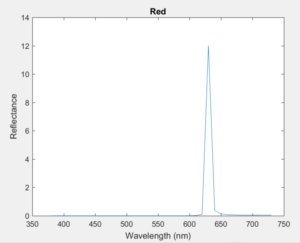green, rgb=(0,1,0)
>> rho=method_2(D, T2020', [EPS,1,EPS]);
>> plot([380:10:730],rho); xlabel('Wavelength (nm)'); ylabel('Reflectance'); title('Green')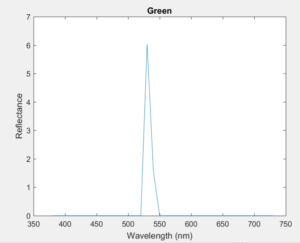blue, rgb=(0,0,1)
>> rho=method_2(D, T2020', [EPS,EPS,1]);
>> plot([380:10:730],rho); xlabel('Wavelength (nm)'); ylabel('Reflectance'); title('Blue')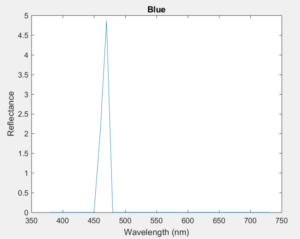cyan, rgb=(0,1,1)
>> rho=method_2(D, T2020', [EPS,1,1]);
>> plot([380:10:730],rho); xlabel('Wavelength (nm)'); ylabel('Reflectance'); title('Cyan')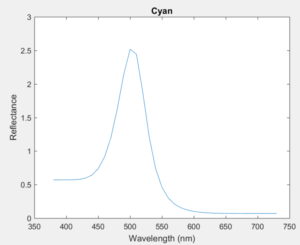magenta, rgb=(1,0,1)
>> rho=method_2(D, T2020', [1,EPS,1]);
>> plot([380:10:730],rho); xlabel('Wavelength (nm)'); ylabel('Reflectance'); title('Magenta')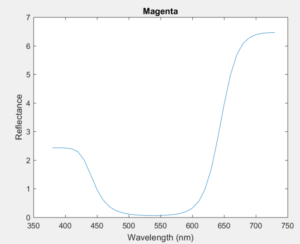yellow, rgb=(1,1,0)
>> rho=method_2(D, T2020', [1,1,EPS]);
>> plot([380:10:730],rho); xlabel('Wavelength (nm)'); ylabel('Reflectance'); title('Yellow')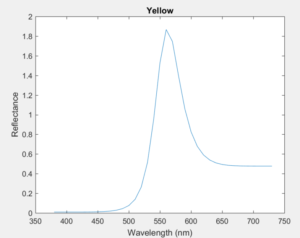Note that the value EPS = 1e-6 is used in place of zero. Method 2 can only produce reflectance curves that are strictly > 0, so this helps with stability of the method.

##### Treating Emissive Sources

You might notice that many of these "reflectance" curves have values greater than one. Normal, non-fluorescent objects can't have reflectances > 1.

Is this a problem? No, not really.

Recall that thematrix is referenced to illuminant D65. That means that a perfect reflector (rho = 1 everywhere) will reflect D65 as a spectral power distribution. Here is D65: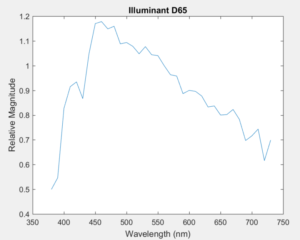The light emitted from the perfect reflector will have this power distribution. The light emitted from an arbitrary object (with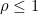) can be computed as diag(D65)*rho (which is an element-by-element multiplication of D65 and rho). For example, an object color rgb = (0.5, 0.2, 0.3) produces the reflectance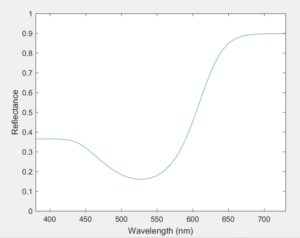The light emitted from the object under D65 is the element-by-element product of the reflectance and the illuminant:This same idea works for "reflectances" that have elements > 1. Just think of them as emissive light sources instead of colored objects. The emissive source would be the product of the "reflectance" and D65. Here, for example, is the emissive source corresponding to cyan, rgb = (0,1,1):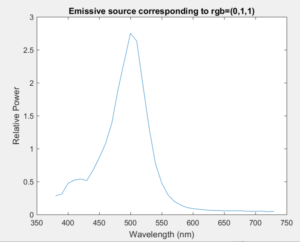So to summarize, when using method 2 to create reflectance reconstructions in Rec. 2020 space, treat colors producing reflectances <=1 as colored objects, and treat colors producing reflectances with elements >1 as emissive sources.

________________________________________

#### Acknowledgments

Thanks to Brien Dieterle, Mark (user kram1032), Chris Cook (user smilebags) and Troy Sobotka (troy_s) on a Blender discussion site for the interesting discussions that prompted me to look into this.

________________________________________

________________________________________